Anúncio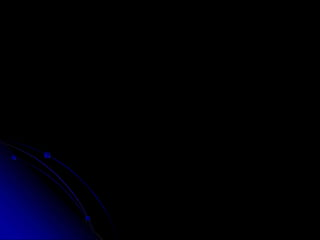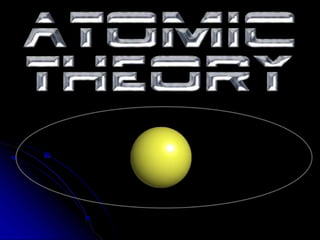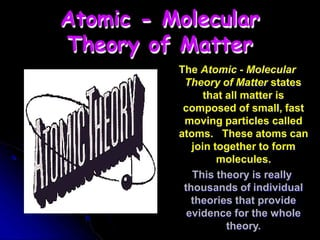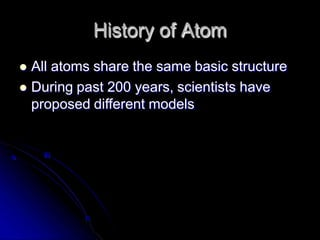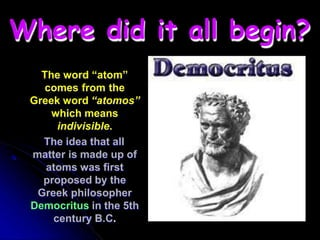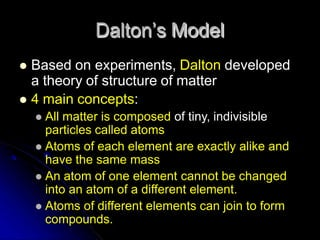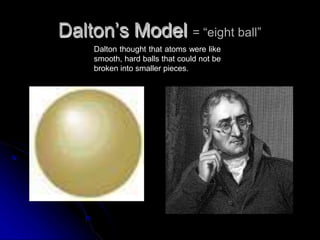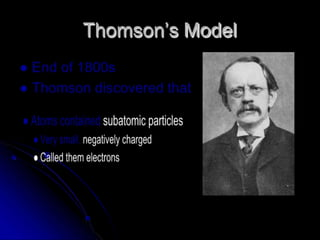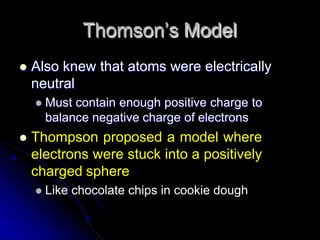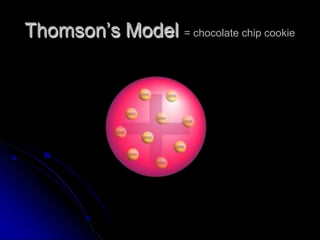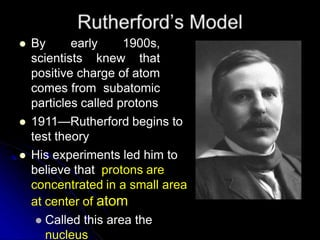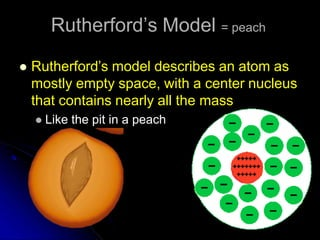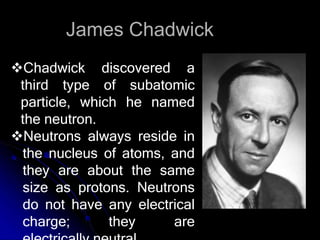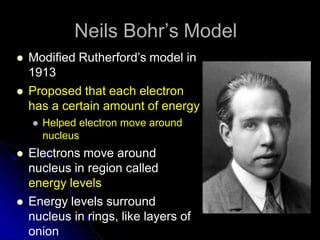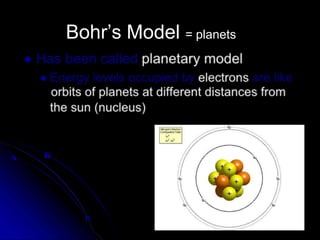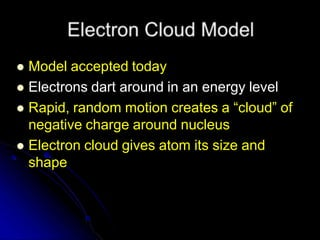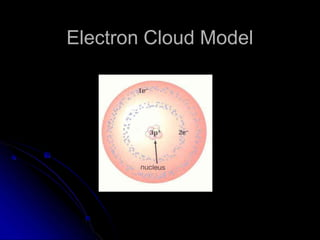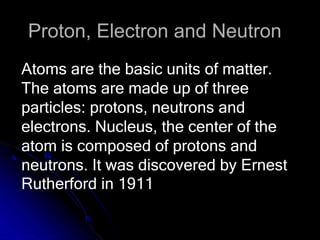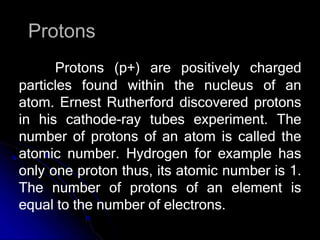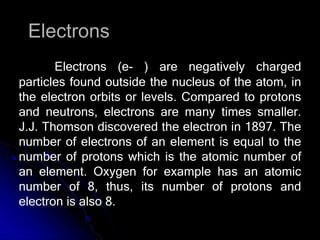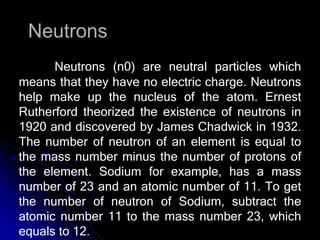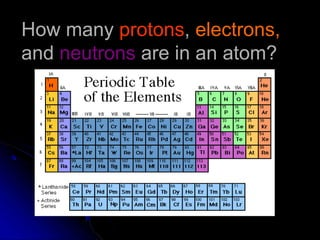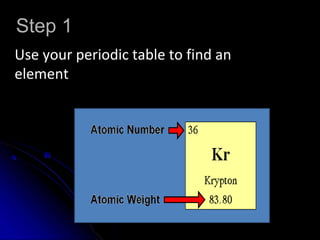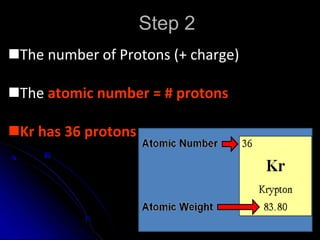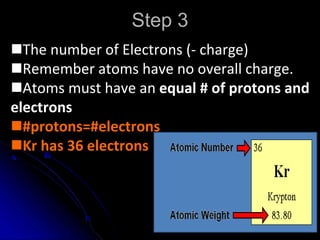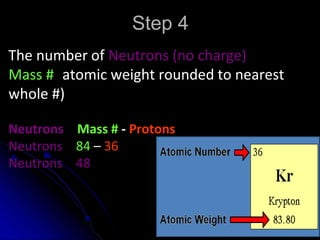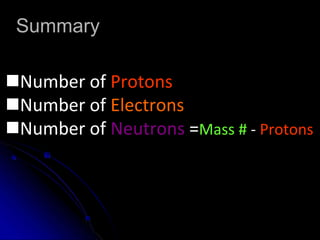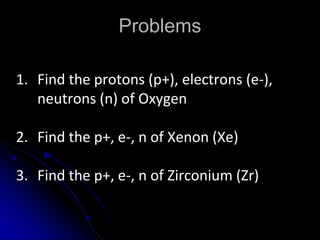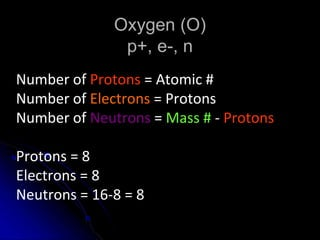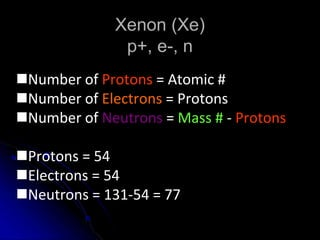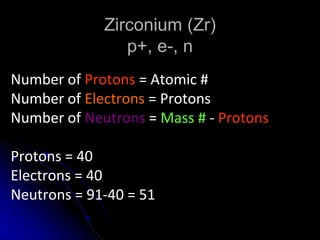1 de 31
Anúncio

### Atomic Theory.pptx

1. Atomic - Molecular Theory of Matter The Atomic - Molecular Theory of Matter states that all matter is composed of small, fast moving particles called atoms. These atoms can join together to form molecules. This theory is really thousands of individual theories that provide evidence for the whole theory.
2. History of Atom  All atoms share the same basic structure  During past 200 years, scientists have proposed different models
3. Where did it all begin? The word “atom” comes from the Greek word “atomos” which means indivisible. The idea that all matter is made up of atoms was first proposed by the Greek philosopher Democritus in the 5th century B.C.
4. Dalton’s Model  Based on experiments, Dalton developed a theory of structure of matter  4 main concepts:  All matter is composed of tiny, indivisible particles called atoms  Atoms of each element are exactly alike and have the same mass  An atom of one element cannot be changed into an atom of a different element.  Atoms of different elements can join to form compounds.
5. Dalton’s Model = “eight ball” Dalton thought that atoms were like smooth, hard balls that could not be broken into smaller pieces.
6. Thomson’s Model
7. Thomson’s Model  Also knew that atoms were electrically neutral  Must contain enough positive charge to balance negative charge of electrons  Thompson proposed a model where electrons were stuck into a positively charged sphere  Like chocolate chips in cookie dough
8. Thomson’s Model = chocolate chip cookie
9.  By early 1900s, scientists knew that positive charge of atom comes from subatomic particles called protons  1911—Rutherford begins to test theory  His experiments led him to believe that protons are concentrated in a small area at center of atom  Called this area the nucleus
10. Rutherford’s Model = peach  Rutherford’s model describes an atom as mostly empty space, with a center nucleus that contains nearly all the mass  Like the pit in a peach
11. James Chadwick Chadwick discovered a third type of subatomic particle, which he named the neutron. Neutrons always reside in the nucleus of atoms, and they are about the same size as protons. Neutrons do not have any electrical charge; they are
12. Neils Bohr’s Model  Modified Rutherford’s model in 1913  Proposed that each electron has a certain amount of energy  Helped electron move around nucleus  Electrons move around nucleus in region called energy levels  Energy levels surround nucleus in rings, like layers of onion
13. Bohr’s Model = planets
14.  Model accepted today  Electrons dart around in an energy level  Rapid, random motion creates a “cloud” of negative charge around nucleus  Electron cloud gives atom its size and shape
15. Electron Cloud Model
16. Proton, Electron and Neutron Atoms are the basic units of matter. The atoms are made up of three particles: protons, neutrons and electrons. Nucleus, the center of the atom is composed of protons and neutrons. It was discovered by Ernest Rutherford in 1911
17. Protons Protons (p+) are positively charged particles found within the nucleus of an atom. Ernest Rutherford discovered protons in his cathode-ray tubes experiment. The number of protons of an atom is called the atomic number. Hydrogen for example has only one proton thus, its atomic number is 1. The number of protons of an element is equal to the number of electrons.
18. Electrons Electrons (e- ) are negatively charged particles found outside the nucleus of the atom, in the electron orbits or levels. Compared to protons and neutrons, electrons are many times smaller. J.J. Thomson discovered the electron in 1897. The number of electrons of an element is equal to the number of protons which is the atomic number of an element. Oxygen for example has an atomic number of 8, thus, its number of protons and electron is also 8.
19. Neutrons Neutrons (n0) are neutral particles which means that they have no electric charge. Neutrons help make up the nucleus of the atom. Ernest Rutherford theorized the existence of neutrons in 1920 and discovered by James Chadwick in 1932. The number of neutron of an element is equal to the mass number minus the number of protons of the element. Sodium for example, has a mass number of 23 and an atomic number of 11. To get the number of neutron of Sodium, subtract the atomic number 11 to the mass number 23, which equals to 12.
20. How many protons, electrons, and neutrons are in an atom?
21. Step 1 Use your periodic table to find an element http://education.jlab.org/qa/pen_number.html
22. Step 2 The number of Protons (+ charge) The atomic number = # protons Kr has 36 protons
23. Step 3 The number of Electrons (- charge) Remember atoms have no overall charge. Atoms must have an equal # of protons and electrons #protons=#electrons Kr has 36 electrons
24. Step 4 The number of Neutrons (no charge) Mass # (atomic weight rounded to nearest whole #) Neutrons = Mass # - Protons Neutrons = 84 – 36 Neutrons = 48
25. Summary Number of Protons = Atomic # Number of Electrons = Protons Number of Neutrons =Mass # - Protons
26. Problems 1. Find the protons (p+), electrons (e-), neutrons (n) of Oxygen 2. Find the p+, e-, n of Xenon (Xe) 3. Find the p+, e-, n of Zirconium (Zr)
27. Oxygen (O) p+, e-, n Number of Protons = Atomic # Number of Electrons = Protons Number of Neutrons = Mass # - Protons Protons = 8 Electrons = 8 Neutrons = 16-8 = 8
28. Xenon (Xe) p+, e-, n Number of Protons = Atomic # Number of Electrons = Protons Number of Neutrons = Mass # - Protons Protons = 54 Electrons = 54 Neutrons = 131-54 = 77
29. Zirconium (Zr) p+, e-, n Number of Protons = Atomic # Number of Electrons = Protons Number of Neutrons = Mass # - Protons Protons = 40 Electrons = 40 Neutrons = 91-40 = 51

### Notas do Editor

1. basic unit of a chemical element.
2. Solid, liquid ang gas composed of atom Molecule is a smallest unit of atom
3. Atoms cannot be subdivided, created, or destroyed.
Anúncio